# Solution assignment 14 Product and Quotient rule

### Assignment 14

Differentiate: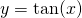### Solution

This function is not in the list of standard functions. However, from trigonometry we know: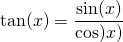and thus we can apply the quotient rule with:and: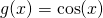and thus: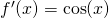and: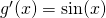and thus the quotient rule yields: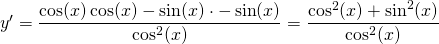We can simplify this formula in two different ways. Because:we can write: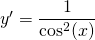Or, when we rewrite the original function we get the following form: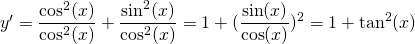0